# Analytic curve

A curvein an-dimensional Euclidean space,, which has an analytic parametrization. This means that the coordinates of its points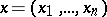can be expressed as analytic functions of a real parameter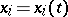,,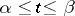, i.e. in a certain neighbourhood of each point,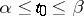, the functionscan be represented as convergent power series in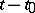, and the derivatives,, do not simultaneously vanish at any point of the segment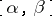. This last condition is sometimes treated separately, and an analytic curve which satisfies it is called a regular analytic curve. An analytic curve is called closed if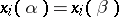,.
An analytic curve in the planeof the complex variable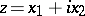can be represented as a complex-analytic function of a real parameter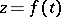,,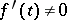on. If the analytic curve is located in a domain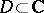, then a conformal mapping ofinto any domain will also yield an analytic curve. If the set of intersection points of two analytic curves is infinite, these analytic curves coincide.
In general, in a complex space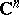,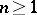, the complex coordinates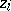of the points of an analytic curve can be represented as analytic functions of a real parameter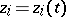,,. It should be noted, however, that if, the term "analytic curve" may sometimes denote an analytic surface of complex dimension one.
On a Riemann surfacean analytic curvecan be represented as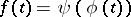, whereis a local uniformizing parameter of the points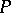onand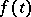is an analytic function of a real parameter in a neighbourhood of any point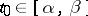.